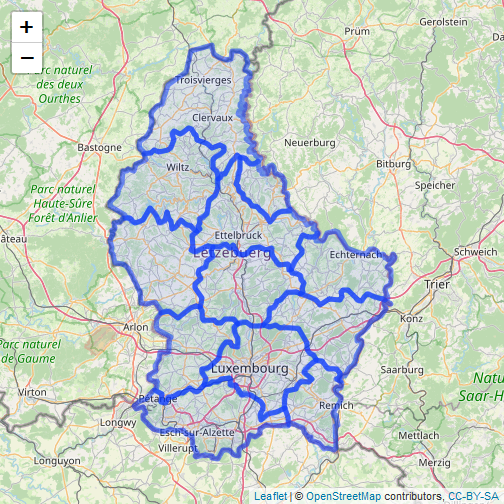# Maps¶

Generally maps are created by running `plot(x)`, sometimes several times.

You can zoom in using `zoom(x)` and clicking on the map twice (to indicate where to zoom to). Or use `select(x)` to save a spatial subset to a new object. With `click(x)` it is possible to interactively query a SpatRaster by clicking once or several times on a map plot.

## SpatVector¶

Example data

```library(terra)
p <- vect(system.file("ex/lux.shp", package="terra"))
```

If you plot a `SpatVector` without further arguments, you get black points, lines or polygons, and no legend.

```plot(p)
```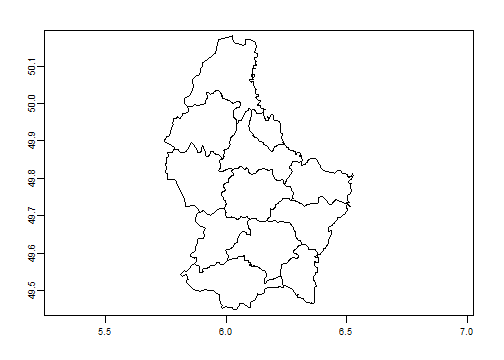You can add colors like this

```n <- nrow(p)
plot(p, col=rainbow(n))
```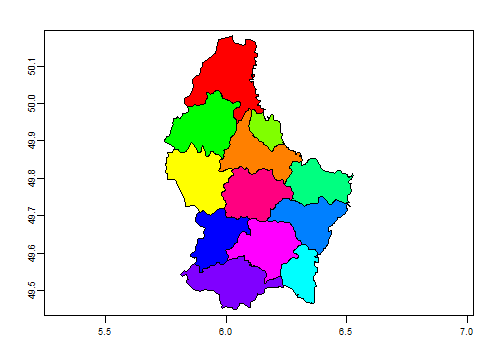But if you want colors it is probably easiest to use an attribute.

```plot(p, "NAME_2", col=rainbow(25))
```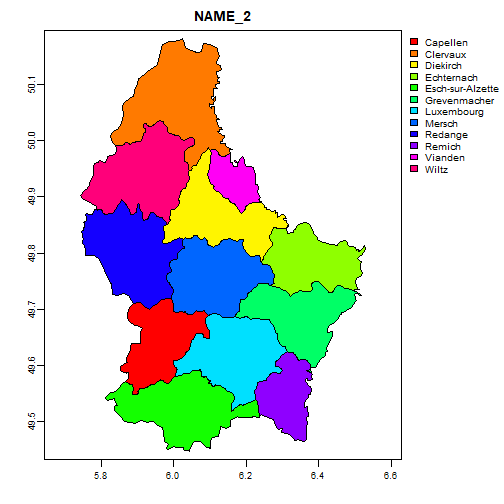You can request maps for multiple variables

```plot(p, c("NAME_1", "NAME_2"), col=rainbow(25))
```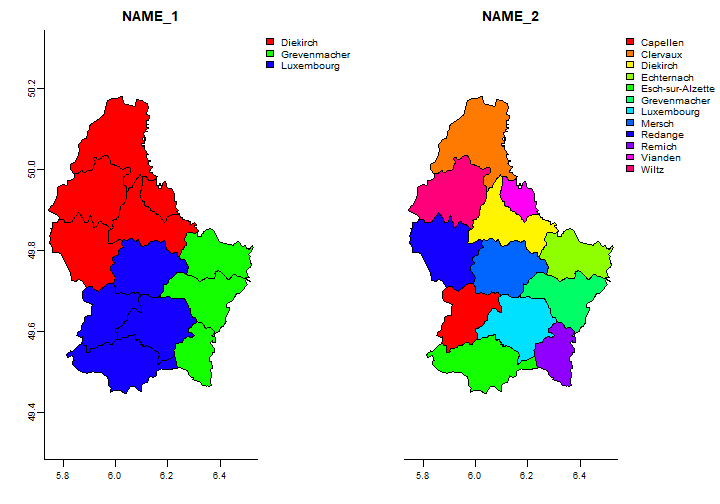Below we also make two maps, but do it “by hand”. We adjust the spacing, and put the legends inside the map area, and use non-rotated text for the vertical axis.

```par(mfrow=c(1,2))
m <- c(3.1, 3.1, 2.1, 2.1)
plot(p, "NAME_1", col=rainbow(25), mar=m, plg=list(x="topright"), pax=list(las=1))
plot(p, "NAME_2", col=rainbow(25), mar=m, plg=list(x="topright", cex=.75), pax=list(las=1))
```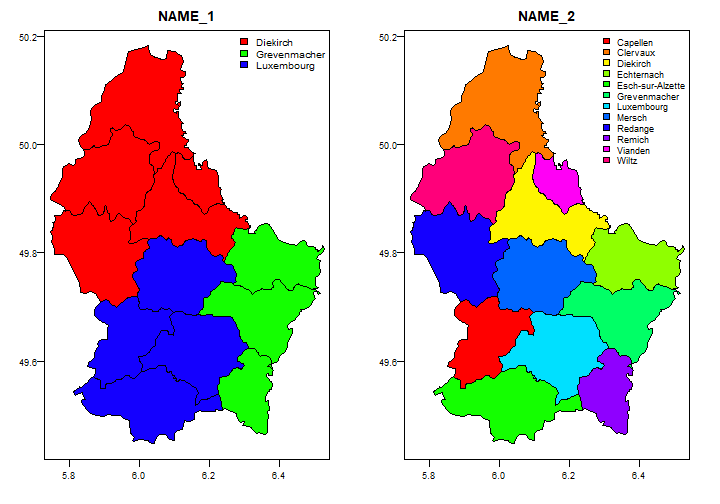More costumization. Choose the axes to draw, at a label and a box to the legend.

```par(mfrow=c(1,2))
m <- c(3.1, 3.1, 1.1, 1.1)
plot(p, "NAME_1", col=rainbow(25), mar=m, plg=list(x="topright", title="District", bty = "o"), main="", axes=FALSE)
axis(1, at=c(5,7)); axis(1)
axis(2, at=c(49,51)); axis(2, las=1)
plot(p, "NAME_2", col=rainbow(25), mar=m, plg=list(x="topright", cex=.75, title="Canton", bty = "o"), main="", axes=FALSE)
axis(1, at=c(5, 7)); axis(1)
```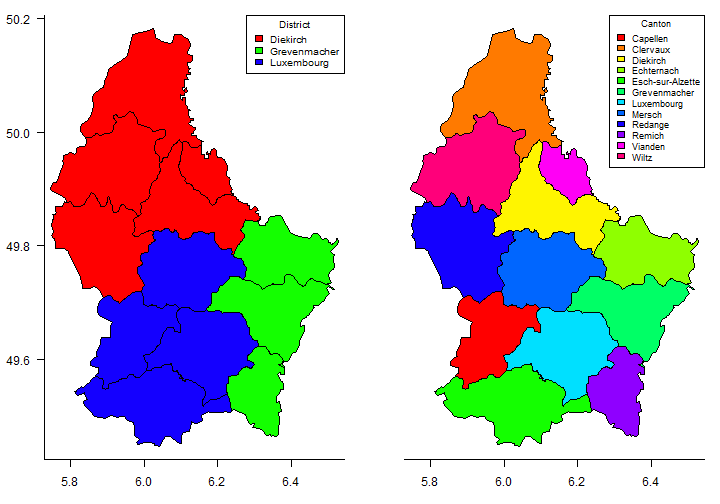We can combine multiple SpatVectors using `lines` and `points` to draw on top of what we plotted first.

```d <- aggregate(p, "NAME_1")
plot(p, col="light blue", lty=2, border="red", lwd=2)
lines(d, lwd=5)
lines(d, col="white", lwd=1)
text(p, "NAME_2", cex=.8, halo=TRUE)
```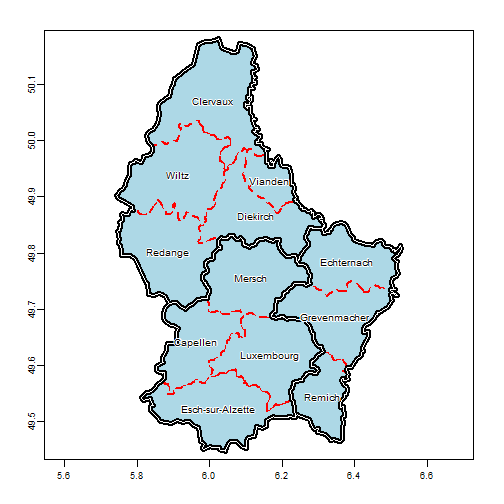The `rasterVis` package provides a lot of very nice plotting options as well.

## SpatRaster¶

Example data

```f <- system.file("ex/elev.tif", package="terra")
r <- rast(f)
```

The default display of a single layer SpatRaster depends on the data type, but there will always be a legend.

```plot(r)
```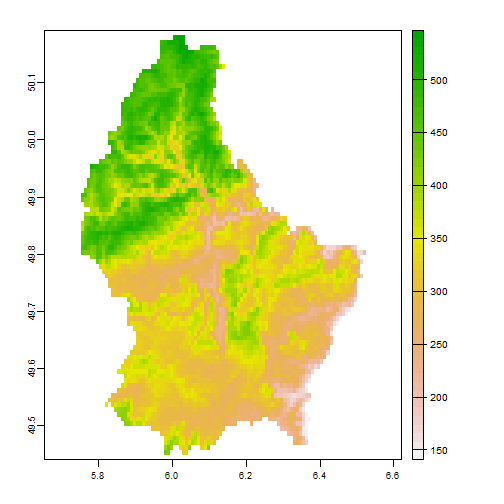After plotting a `SpatRaster` you can add vector type spatial data (points, lines, polygons). You can do this with functions `points`, `lines`, `polys` or `plot(object, add=TRUE)`.

```plot(r)
lines(p, lwd=2)
set.seed(12)
xy <- spatSample(r, 20, "random", na.rm=TRUE, xy=TRUE)
points(xy, pch=20, col="red", cex=2)
```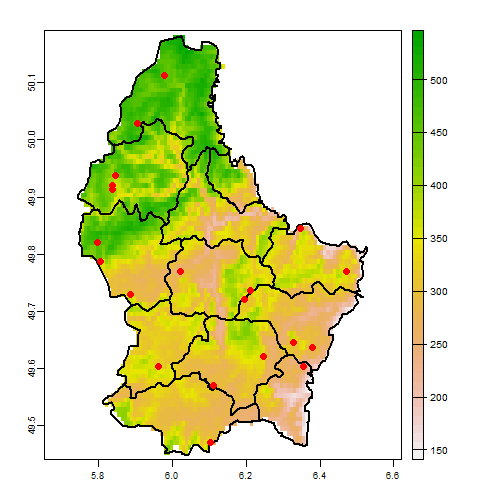Or use a different legend type

```m <- c(3.1, 3.1, 1.1, 1.1)
plot(r, type="interval", plg=list(x="topright"), mar=m)
```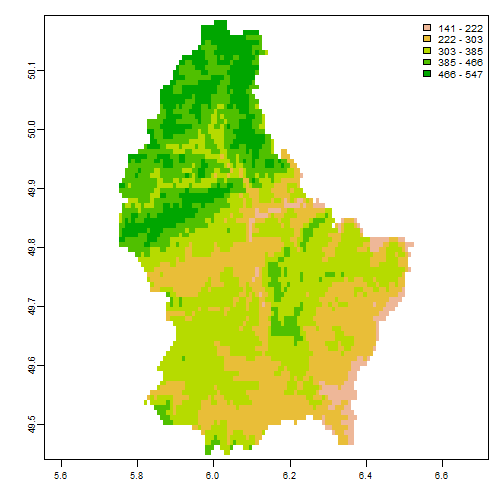If there are only a few values, the default is to show “classes”

```rr <- round(r/100)
plot(rr, plg=list(x="topright"), mar=m)
```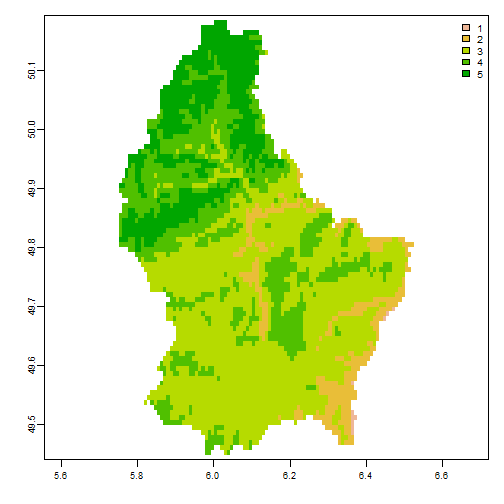If the raster is categorical you get the labels

```set.seed(0)
x <- rast(nrow=10, ncol=10)
values(x) <- sample(0:2, ncell(r), replace=TRUE)
## Warning: [setValues] length of values does not match the number of cells
cls <- c("forest", "water", "urban")
levels(x) <- cls
names(x) <- "land cover"
is.factor(x)
##  TRUE
x
## class       : SpatRaster
## dimensions  : 10, 10, 1  (nrow, ncol, nlyr)
## resolution  : 36, 18  (x, y)
## extent      : -180, 180, -90, 90  (xmin, xmax, ymin, ymax)
## coord. ref. : +proj=longlat +datum=WGS84 +no_defs
## source      : memory
## name        : land cover
## min value   :     forest
## max value   :      water
plot(x, col=c("green", "blue", "light gray"))
text(x, digits=3, cex=.75, halo=TRUE)
```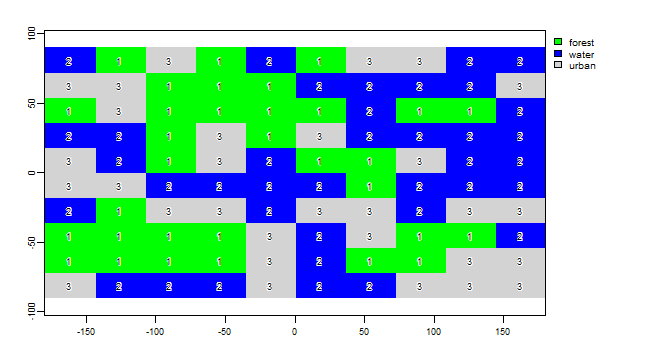When plot is used with a multi-layer object, all layers are plotted (up to 16), unless the layers desired are indicated with an additional argument.

```library(terra)
b <- rast(system.file("ex/logo.tif", package="terra"))
plot(b)
``````r <- rast(p, res=0.01 )
values(r) <- 1:ncell(r)
```

In this case, it makes sense to combine the three layers into a single image, by assigning individual layers to one of the three color channels (red, green and blue):

```plotRGB(b, r=1, g=2, b=3)
```You can also use a number of other plotting functions with `SpatRasters`, including `hist`, `persp`, `contour`}, and `density`. See the help files for more info.

The `rasterVis` and `tmap` packages provides a lot of very nice mapping options as well.

## Basemaps¶

You can get many different basemaps with the maptiles package. Reading the data again.

```library(terra)
f <- system.file("ex/lux.shp", package="terra")
p <- vect(f)
```
```library(maptiles)
bg <- get_tiles(ext(p))
plotRGB(bg)
lines(p, col="blue", lwd=3)
```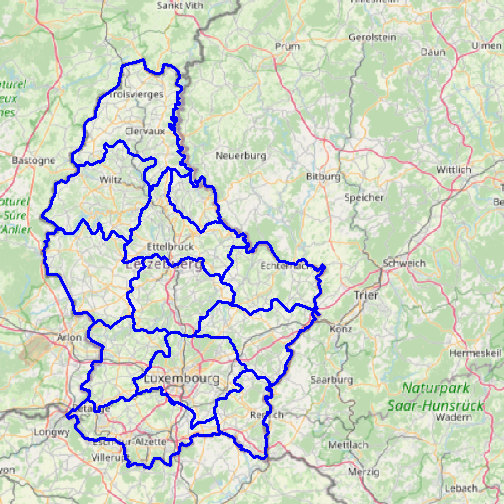## Interactive maps¶

You can use the `leaflet` package to make interactive maps.

```library(sf)
library(leaflet)
leaflet(sf::st_as_sf(p)) %>%